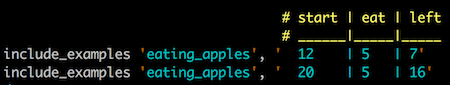## Wednesday, June 28, 2017

### Scenario Outline in RSpec

Some business logic is best described in a table format. The rows and columns can better detail all the different permutations, than words and sentences. Gherkin handles this well with its scenario outlines, however, RSpec does not have such feature.

As I wanted to test all the different permutation of a business logic, I ended up with a ~200 lines spec file. It was hard to review what use cases were covered as I had to scroll up and down to review the file. Had RSpec have a table format, this logic could have been described in 40 lines, which can easily fit into a screen.

It was obvious a simple DSL was needed, but what would that look like? This is what I came up with first:

```RSpec.describe 'eating apples' do
def method_under_test(start, eat, left)
start - eat == left
end

def example_runner(examples)
lines = examples.split(/\n/)
lines.delete_at(0) # Don't need the title
lines.each do |line|
example = line.split('|')
start, eat, left = example.reject(&:blank?).map(&:to_i)
expect(method_under_test(start, eat, left)).to eq(true)
end
end

it 'can tell how many are remaining' do
examples =
<<~TABLE
| start | eat | left |
| 12    | 5   | 7    |
| 20    | 5   | 15   |
TABLE

example_runner(examples)
end
end
```

I am using the `example_runner` method to parse the examples table and run the specs one by one. The spec itself is clear, the examples TABLE is easy to visualize.

The spec documentation, however, does not reveal the used table. This is what it looks like:

```eating apples
can tell how many are remaining
```

Let’s say, one of the examples will trigger an error. When I change the second example’s “left” column’s 15 value to 16, this is what I see when I run the spec.

```F

Failures:

1) eating apples can tell how many are remaining
Failure/Error: expect(method_under_test(start, eat, left)).to eq(true)

expected: true
got: false

(compared using ==)
```

This is not very useful. I don’t know which example failed. Fortunately RSpec lets us provide more context for an expectation. When I change the line of the assertion with an added context like this,

```expect(method_under_test(start, eat, left)).to eq(true), line
```

the example that failed is properly reported by RSpec:

```F

Failures:

1) eating apples can tell how many are remaining
Failure/Error: expect(method_under_test(start, eat, left)).to eq(true), line
| 20    | 5   | 16   |
```

This could be a decent solution, however, not printing the examples for the documentation output has major drawbacks. Every row in the table should be a separate example, it should be expressed as such. With some clever formatting and using `include_example` a similar table layout can be achieved. Consider this example:

```RSpec.describe 'eating apples' do
def method_under_test(start, eat, left)
start - eat == left
end

RSpec.shared_examples 'eating_apples' do |example|
it "calculates the remaining apple for - #{example}" do
parsed_example = example.split('|')
start, eat, left = parsed_example.reject(&:blank?).map(&:to_i)

result = method_under_test(start, eat, left)
expect(result).to eq(true)
end
end

# start | eat | left
# ______|_____|_____
include_examples 'eating_apples', '  12    | 5   | 7'
include_examples 'eating_apples', '  20    | 5   | 15'
end
```

The table is shifted to the right, but it’s clearly visible, and the commented out header provides a different color in my IDE that separates it from the data.The `shared_example` is also a lot simpler, I don’t need to deal with the header information, as it’s not passed to it, examples are being ran line by line.

By making the test description dynamic, the reporter prints out the tested values:

```eating apples
calculates the remaining apple for -   12    | 5   | 7
calculates the remaining apple for -   20    | 5   | 15
```

The triggered error is properly reported by both the line number and the example used for that particular use case.

```.F

Failures:

1) eating apples calculates the remaining apple for -   20    | 5   | 16
Failure/Error: expect(result).to eq(true)

expected: true
got: false

(compared using ==)
```

I am not a big fan of adjusted white space for positioning equal signs under one another, but am happy to use white space to format test code to better describe the logic it validates.

RSpec does not have scenario outlines, but hopefully, with a `shared_example` and a bit of clever formatting the same can be accomplished.